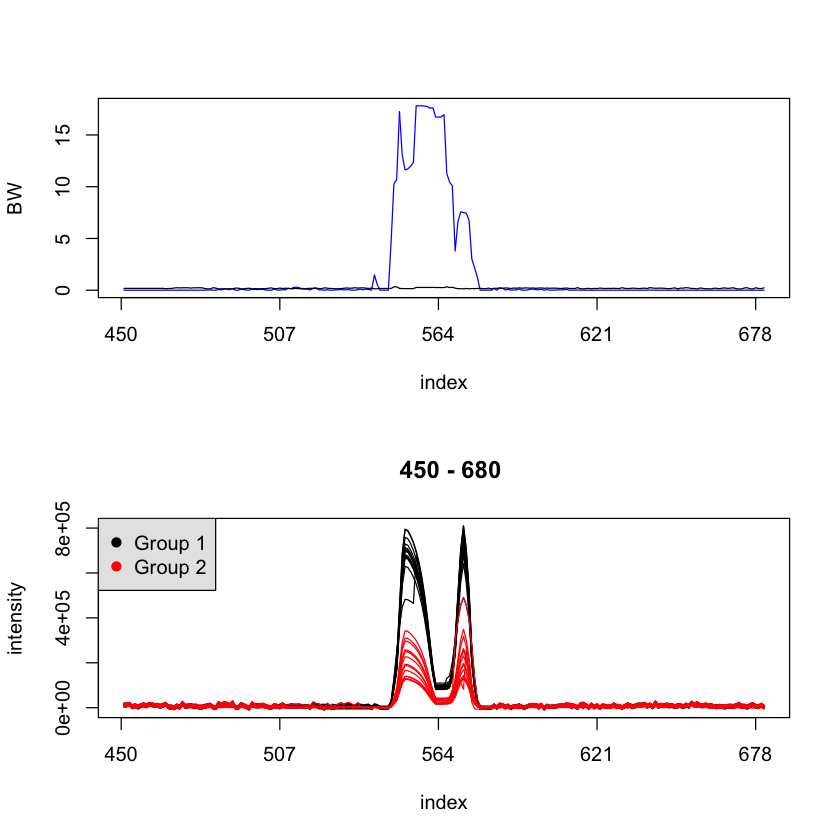# User guide for speaq package version <= 1.2.3

## Preface

This introduction was written for the speaq package up until version 1.2.3. Since version 2.0 a lot of functionality is added but the original functionality is maintained. This vignette can therefor still be used as it describes one part of the package dealing with spectral alignment and quantitation.

## Introduction

We introduce a novel suite of informatics tools for the quantitative analysis of NMR metabolomic profile data. The core of the processing cascade is a novel peak alignment algorithm, called hierarchical Cluster-based Peak Alignment (CluPA).

The algorithm aligns a target spectrum to the reference spectrum in a top-down fashion by building a hierarchical cluster tree from peak lists of reference and target spectra and then dividing the spectra into smaller segments based on the most distant clusters of the tree. To reduce the computational time to estimate the spectral misalignment, the method makes use of Fast Fourier Transformation (FFT) cross-correlation. Since the method returns a high-quality alignment, we can propose a simple methodology to study the variability of the NMR spectra. For each aligned NMR data point the ratio of the between-group and within-group sum of squares (BW-ratio) is calculated to quantify the difference in variability between and within predefined groups of NMR spectra. This differential analysis is related to the calculation of the F-statistic or a one-way ANOVA, but without distributional assumptions. Statistical inference based on the BW-ratio is achieved by bootstrapping the null distribution from the experimental data.

We are going to introduce step-by-step how part of speaq works for a specific dataset, this includes

• automatically do alignment
• allow user intervening into the process
• compute BW ratios
• visualize results

For any issue reports or discussions about speaq feel free to contact us via the developing website at github (https://github.com/beirnaert/speaq).

## Data input

We randomly generate an NMR spectral dataset of two different groups (15 spectra for each group). Each spectrum has two peaks slightly shifted cross over spectra. More details are described in the manual document of function makeSimulatedData().

library(speaq)
#Generate a simulated NMR data set for this experiment
res=makeSimulatedData();
X=res$data; groupLabel=res$label;

Now, we draw a spectral plot to observe the dataset before alignment.

drawSpec(X)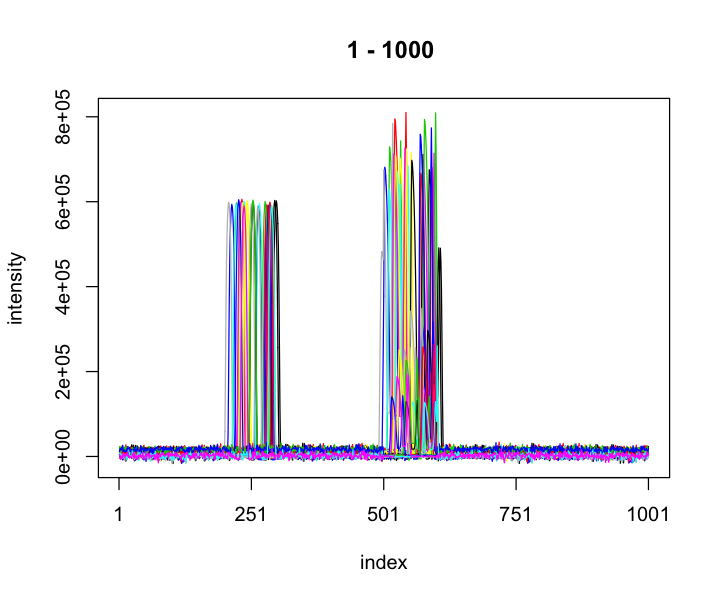## Landmark peak detection

This section makes use of MassSpecWavelet package to detect peak lists of the dataset.

cat("\n detect peaks....")
##
##  detect peaks....
startTime <- proc.time()
peakList <- detectSpecPeaks(X, nDivRange = c(128), scales = seq(1, 16, 2), baselineThresh = 50000,
SNR.Th = -1, verbose = FALSE)

endTime <- proc.time()
cat("Peak detection time:", (endTime - startTime)/60, " minutes")
## Peak detection time: 0.02146667  minutes

## Reference finding

Next, We find the reference for other spectra align to.

cat("\n Find the spectrum reference...")
##
##  Find the spectrum reference...
resFindRef <- findRef(peakList)
refInd <- resFindRef$refInd # The ranks of spectra for (i in seq_along(resFindRef$orderSpec)) {
cat(paste(i, ":", resFindReforderSpec[i], sep = ""), " ") if (i%%10 == 0) cat("\n") } ## 1:24 2:25 3:27 4:9 5:21 6:16 7:7 8:23 9:19 10:18 ## 11:5 12:30 13:12 14:10 15:15 16:20 17:2 18:6 19:22 20:26 ## 21:14 22:11 23:29 24:28 25:3 26:13 27:17 28:1 29:4 30:8 cat("\n The reference is: ", refInd) ## ## The reference is: 24 ## Spectral alignment For spectral alignment, function dohCluster() is used to implement hierarchical Cluster-based Peak Alignment  (CluPA) algorithm. In this function maxShift is set by 100 by default which is suitable with many NMR datasets. Experienced users can set select more proper for their dataset. For example: # Set maxShift maxShift = 50 Y <- dohCluster(X, peakList = peakList, refInd = refInd, maxShift = maxShift, acceptLostPeak = TRUE, verbose = FALSE) ### Automatically detect the optimal maxShift If users are not confident when selecting a value for the maxShift, just set the value to NULL. Then, the software will automatically learn to select the optimal value based on the median Pearson correlation coefficient between spectra. It is worth noting that this metric is significantly effected by high peaks in the spectra , so it might not be the best measure for evaluating alignment performances. However, it is fast for the purpose of detecting the suitable maxShift value. This mode also takes more time since CluPA implements extra alignment for few maxShift values. If set verbose=TRUE, a plot of performances of CluPA with different values of maxShift will be displayed. For example: Y <- dohCluster(X, peakList = peakList, refInd = refInd, maxShift = NULL, acceptLostPeak = TRUE, verbose = TRUE) ## ## -------------------------------- ## maxShift=NULL, thus CluPA will automatically detect the optimal value of maxShift. ## -------------------------------- ## ## maxShift= 2 ## Median Pearson correlation coefficent: -0.02429686 , the best result: -1 ## maxShift= 4 ## Median Pearson correlation coefficent: 0.006180729 , the best result: -0.02429686 ## maxShift= 8 ## Median Pearson correlation coefficent: 0.0452733 , the best result: 0.006180729 ## maxShift= 16 ## Median Pearson correlation coefficent: 0.7615448 , the best result: 0.0452733 ## maxShift= 32 ## Median Pearson correlation coefficent: 0.9317428 , the best result: 0.7615448 ## maxShift= 64 ## Median Pearson correlation coefficent: 0.9317428 , the best result: 0.9317428 ## maxShift= 128 ## Median Pearson correlation coefficent: 0.9317428 , the best result: 0.9317428 ## maxShift= 256 ## Median Pearson correlation coefficent: 0.9317428 , the best result: 0.9317428 ## Optimal maxShift= 32 with median Pearson correlation of aligned spectra= 0.9317428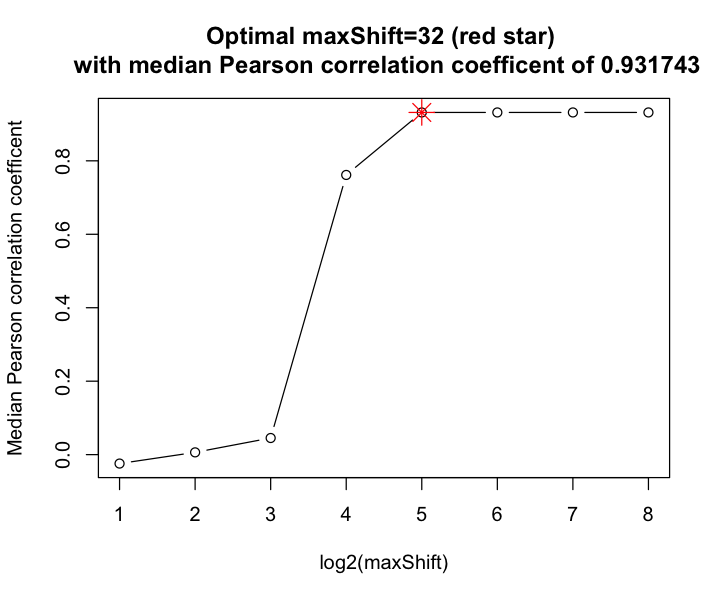## ## Alignment time: 0.008383333 minutes In this example, the best maxShift=32 which is highlighted by a red star in the plot achieves the highest median Pearson correlation coefficient (0.93). ### Spectral alignment with selected segments If users just want to align in specific segments or prefer to use different parameter settings for different segments. speaq allows users to do that by intervene into the process. To do that, users need to create a segment information matrix as the example in Table 1. begin end forAlign ref maxShift 100 200 0 0 0 450 680 1 0 50 Each row contains the following information corresponding to the columns: • begin: the starting point of the segment. • end: the end point of the segment. • forAlign: the segment is aligned (1) or not (0). • ref: the index of the reference spectrum. If 0, the algorithm will select the reference found by the reference finding step. • maxShift: the maximum number of points of a shift to left/right. It is worth to note that only segments with forAlign=1 (column 3) will be taken into account for spectral alignment. Now, simply run dohClusterCustommedSegments with the input from the information file. segmentInfoMat = matrix(data = c(100, 200, 0, 0, 0, 450, 680, 1, 0, 50), nrow = 2, ncol = 5, byrow = TRUE) colnames(segmentInfoMat) = c("begin", "end", "forAlign", "ref", "maxShift") segmentInfoMat ## begin end forAlign ref maxShift ## [1,] 100 200 0 0 0 ## [2,] 450 680 1 0 50 Yc <- dohClusterCustommedSegments(X, peakList = peakList, refInd = refInd, segmentInfoMat = segmentInfoMat, minSegSize = 128, verbose = FALSE) ## Spectral plots We could draw a segment to see the performance of the alignment. drawSpec(Y)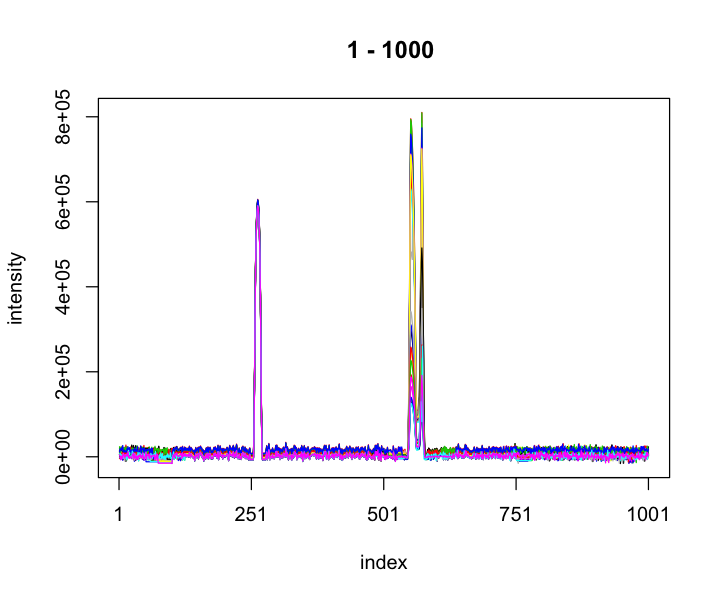We could limit the heights of spectra to easily check the alignment performance. drawSpec(Y, startP = 450, endP = 680, highBound = 5e+05, lowBound = -100)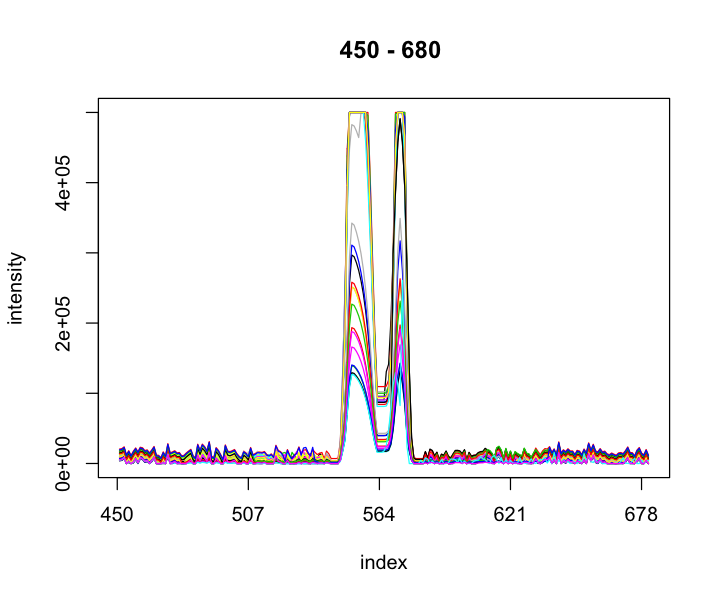We achieved similar results with Yc but the region of the first peak was not aligned because the segment information just allows align the region 450-680. drawSpec(Yc)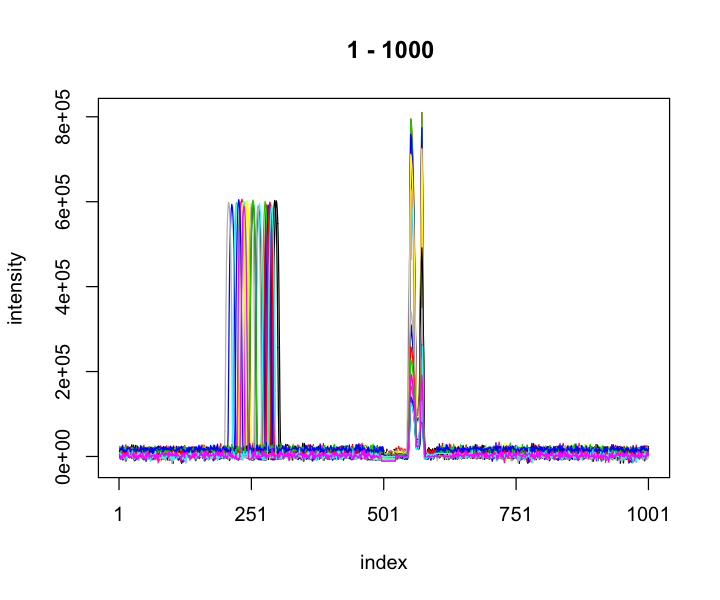## Quantitative analysis This section presents the quantitative analysis for wine data that was used in our paper . To save time, we just do permutation 100 times to create null distribution. N = 100 alpha = 0.05 # find the BW-statistic BW = BWR(Y, groupLabel) # create sampled H0 and export to file H0 = createNullSampling(Y, groupLabel, N = N, verbose = FALSE) # compute percentile of alpha perc = double(ncol(Y)) alpha_corr = alpha/sum(returnLocalMaxima(Y[2, ])pkMax > 50000)
for (i in seq_along(perc)) {
perc[i] = quantile(H0[, i], 1 - alpha_corr, type = 3)
}

Now, some figures are plotting. Read the publication to understand more about these figures.

drawBW(BW, perc, Y, groupLabel = groupLabel)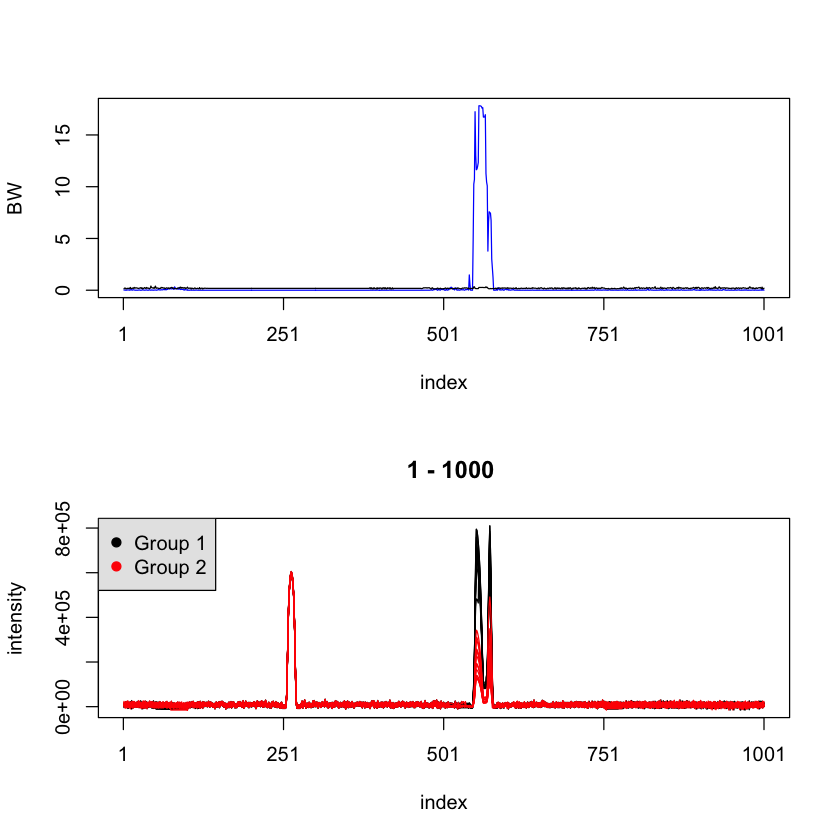drawBW(BW, perc, Y, startP = 450, endP = 680, groupLabel = groupLabel)